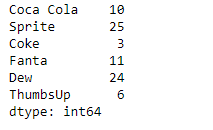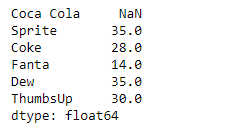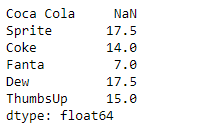Related Articles

# Python | Pandas Series.rolling()

• Last Updated : 07 Feb, 2019

Pandas series is a One-dimensional ndarray with axis labels. The labels need not be unique but must be a hashable type. The object supports both integer and label-based indexing and provides a host of methods for performing operations involving the index.

Pandas` Series.rolling()` function is a very useful function. It Provides rolling window calculations over the underlying data in the given Series object.

Syntax: Series.rolling(window, min_periods=None, center=False, win_type=None, on=None, axis=0, closed=None)

Parameter :
window : Size of the moving window
min_periods : Minimum number of observations in window required to have a value
center : Set the labels at the center of the window.
win_type : Provide a window type.
on : str, optional
axis : int or str, default 0
closed : Make the interval closed on the ‘right’, ‘left’, ‘both’ or ‘neither’ endpoints.

Returns : a Window or Rolling sub-classed for the particular operation

Example #1: Use `Series.rolling()` function to find the rolling window sum of the underlying data for the given Series object. The size of the rolling window should be 2 and the weightage of each element should be same.

 `# importing pandas as pd``import` `pandas as pd`` ` `# Creating the Series``sr ``=` `pd.Series([``10``, ``25``, ``3``, ``11``, ``24``, ``6``])`` ` `# Create the Index``index_ ``=` `[``'Coca Cola'``, ``'Sprite'``, ``'Coke'``, ``'Fanta'``, ``'Dew'``, ``'ThumbsUp'``]`` ` `# set the index``sr.index ``=` `index_`` ` `# Print the series``print``(sr)`

Output :Now we will use `Series.rolling()` function to find the sum of the underlying data having a window size of 2.

 `# Find sum over a window size of 2``result ``=` `sr.rolling(``2``).``sum``()`` ` `# Print the returned Series object``print``(result)`

Output :As we can see in the output, the `Series.rolling()` function has successfully returned a series object having found the sum of the underlying data over a window size of 2. Notice the first value is a missing value as there was no element previous to it so the sum could not be performed.

Example #2: Use `Series.rolling()` function to find the rolling window sum of the underlying data for the given Series object. The size of the rolling window should be 2 and the rolling window type should be ‘triang’.

 `# importing pandas as pd``import` `pandas as pd`` ` `# Creating the Series``sr ``=` `pd.Series([``10``, ``25``, ``3``, ``11``, ``24``, ``6``])`` ` `# Create the Index``index_ ``=` `[``'Coca Cola'``, ``'Sprite'``, ``'Coke'``, ``'Fanta'``, ``'Dew'``, ``'ThumbsUp'``]`` ` `# set the index``sr.index ``=` `index_`` ` `# Print the series``print``(sr)`

Output :Now we will use `Series.rolling()` function to find the sum of the underlying data having a window size of 2.

 `# Find sum over a window size of 2``# We have also provided the window type``result ``=` `sr.rolling(``2``, win_type ``=``'triang'``).``sum``()`` ` `# Print the returned Series object``print``(result)`

Output :As we can see in the output, the `Series.rolling()` function has successfully returned a series object having found the sum of the underlying data over a window size of 2. Notice the first value is a missing value as there was no element previous to it so the sum could not be performed.

Attention geek! Strengthen your foundations with the Python Programming Foundation Course and learn the basics.

To begin with, your interview preparations Enhance your Data Structures concepts with the Python DS Course. And to begin with your Machine Learning Journey, join the Machine Learning – Basic Level Course

My Personal Notes arrow_drop_up